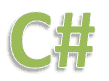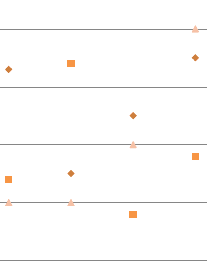# String to byte Array

## Convert String to ByteArrayA String can be converted to Byte Array in few different ways. In .Net, every string has a character set and encoding. Encoding is the process of transforming a set of Unicode characters into a sequence of bytes.When you try to convert a String object to Byte Array, you still have a character set and encoding and it depends on the encoding of your string whether its is in ASCII or UTF8. So you can use System.Text.Encoding.Unicode.GetBytes() to retrieve the set of bytes that Microsoft.Net would using to represent the characters.

### Using UTF8 conversion

`byte[] bArray = Encoding.UTF8.GetBytes (inputString);`

### Using ASCII conversion

`byte[] bArray = Encoding.ASCII.GetBytes (inputString);`
UTF8 Example
```using System;
using System.Collections.Generic;
using System.Linq;
using System.Text;
namespace ConsoleApplication1
{
class Program
{
static void Main(string[] args)
{
const string inputSring = "Halo World";
byte[] bArray = Encoding.UTF8.GetBytes(inputSring);
foreach (byte bElements in bArray)
{
Console.WriteLine("{0} = {1}", (char)bElements, bElements);
}
}
}
}
```
Output:
```H=72
a=97
l=108
o=111
= 32
W=87
o=111
r=114
l=108
d=100
```
ASCII Example
```using System;
using System.Collections.Generic;
using System.Linq;
using System.Text;
namespace ConsoleApplication1
{
class Program
{
static void Main(string[] args)
{
const string inputSring = "Halo World";
byte[] bArray = Encoding.ASCII.GetBytes(inputSring);
foreach (byte bElements in bArray)
{
Console.WriteLine("{0} = {1}",  (char)bElements,  bElements);
}
}
}
}
```
```H=72
a=97
l=108
o=111
= 32
W=87
o=111
r=114
l=108
d=100
```

### Note:You need to take the encoding into account, because one character could be represented by one or more bytes (it may be up to about 6), and different encodings will treat these bytes in a different way. Strings are stored with two bytes per character. ASCII only allows one byte per character.

NEXT.....Detecting arrow keys